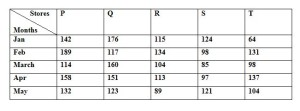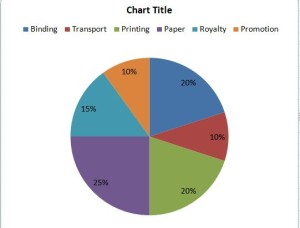#Quants Questions : Data Interpretation Set 14

Hello Aspirants. Welcome to Online Quantitative Aptitude section in AffairsCloud.com. Here we are creating question sample From Data Intrepretation that is important for IBPS,SBI exam,SSC exam, Railways Exam,FCI exam  and other competitive exams. We have included Some questions that are repeatedly asked in exams !!

I. Study the given information to answer the question given below
Number of Munch sold by 5 stores during 5 months1. Number of Munch sold by store T in Jan is what percentage less than the number of Munch sold by store Q March ?
A)40%
B)50%
C)60%
D)64%
E)None of these
C)60%
Explanation :(160 – 64)*100/160 = 60%

2. What is the average number of Munch sold by store R in last four months ?
A)110
B)112
C)120
D)210
E)None of these
A)110
Explanation :(134+104+113+89 )/4 = 110

3. What is the ratio between total number of Munch sold by Store P and Q together in April and the total number of Munch sold by store R and S in the same month ?
A)101:56
B)102:60
C)70:103
D)103:70
E)None of these
D)103:70
Explanation :(158+151) : (113+97) = 309:210 = 103:70

4. What is the difference between the total no of Munch sold by store Q and R together in May to the total number of Munch sold by store P and S in March ?
A)11
B)12
C)13
D)14
E)None of these
C)13
Explanation :(123+89) – (114+85) = 212 – 199 = 13

5. The   maximum number of Munch sold by which store and in which month ?
A)Mar, P
B)Mar, Q
C)Jan, Q
D)Feb, P
E)None of these
D)Feb, P

II. Study the given information to answer the question given below1. If 500 copies are published and the transportation cost on the amount to Rs.2,500 then what should be the selling price of the book so that the publisher can earn a profit of 25%
A)Rs.62.5
B) Rs.65.2
C) Rs.62.2
D) Rs.65.5
E)None of these
A)Rs.62.5
Explanation :
10% = 2500
125% = 125*2500/10 = 31250
Sp of 1 book = 31250/500 =62.5

2. If for an edition of the book, the cost of the paper is Rs.6250, then find the promotion cost for this edition ?
A)Rs.2300
B) Rs.4300
C) Rs.2500
D) Rs.2200
E)None of these
C) Rs.2500
Explanation :
25% = 6250
10% = 10*6250/25 = 2500

3. Find the degree corresponding to the Royalty
A)45degree
B)23degree
C)54degree
D)45degree
E)None of these
C)54degree
Explanation :
R = 15%
Angle = (360*15)/100 = 54degree

4. Which two expenditure together have central angle of144degree
A)Binding And Promotion
B)Transport And Binding
C)Paper And Royalty
D)Paper And Transport
E)None Of These
C)Paper And Royalty
Explanation :
(x*360)/100 = 144
X = 144*100/360 = 40% = 25%(paper) + 15%(Royalty)

5. Find the total cost to publish 350 book if binding cost is Rs.165
A)3,89,000
B)3,98,500
C)3,58,000
D)3,40,500
E)2,88,750
E)2,88,750
Explanation :
20% = 165
100% = (100*165)/20 = 825
350 book = 825*350 = 2,88,750

AffairsCloud Recommends Oliveboard Mock Test

AffairsCloud Ebook - Support Us to Grow